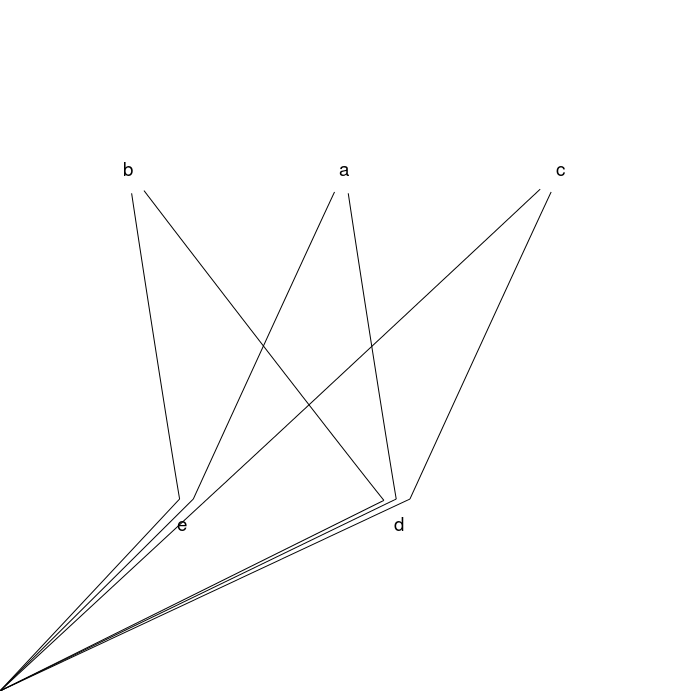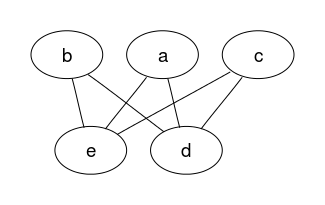# Example 7: subgraphs

An directed graph with 5 nodes, 5 edges, and two labelled subgraphs.

## Graph declaration

In all cases the graph is explicitly declared with ellipse shaped nodes. The dot file edges are declared in alphabetical order as this is the (assumed) default for how Rgraphviz will pass edges internally to graphviz.

 Dot file for graphviz: `````` graph { node [shape=ellipse]; splines=line; subgraph cluster_0 { label="Subgraph A"; a; b; c } subgraph cluster_1 { label="Subgraph B"; d; e; } a -- d; a -- e; b -- d; b -- e; c -- d; c -- e; } `````` R code for Rgraphviz and gridGraphviz: ```# load Rgraphviz library library(Rgraphviz) # nodes nodes <- letters[1:5] # edges edgeList <- list(a=list(edges=c("d","e")), b=list(edges=c("d","e")), c=list(edges=c("d","e")), d=list(edges=c("a","b","c")), e=list(edges=c("a","b","c"))) # graph graph <- new("graphNEL", nodes=nodes, edgeL=edgeList, edgemode="undirected") # subgraphs sgL <- list() sg1 <- subGraph(letters[1:3], graph) sg2 <- subGraph(letters[4:5], graph) sgL[] <- list(graph=sg1, attrs = c(label = "Subgraph A")) sgL[] <- list(graph=sg2, attrs = c(label = "Subgraph B")) ```

## Graph rendering:

### gridGraphviz (new)

``````>> dot -Tpng ex7.dot -o ex7.png
`````````# create Ragraph object
rag <- agopen(graph, "", subGList=sgL,
attrs=list(node=list(shape="ellipse"),
graph=list(splines="line")))
```
```#plot
plot(rag)
``````library(gridGraphviz)

# create Ragraph object
rag <- agopen(graph, "", subGList=sgL,
attrs=list(node=list(shape="ellipse"),
graph=list(splines="line")))
```
```#plot
grid.graph(rag)
```
```## Warning: Unsupported node shape; using 'box'
## Warning: Unsupported node shape; using 'box'
## Warning: Unsupported node shape; using 'box'
## Warning: Unsupported node shape; using 'box'
## Warning: Unsupported node shape; using 'box'
``````library(gridGraphviz)

# create Ragraph object
rag <- agopenTrue(graph, "", subGList=sgL,
attrs=list(node=list(shape="ellipse"),
graph=list(splines="line")))
```
```#plot
grid.graph(rag)
```## Discussion

gridGraphviz (old) fails to produce ellipse shaped nodes, does not label or subgraphs, or place inside boxes.